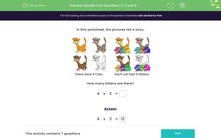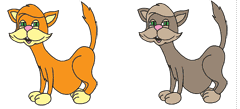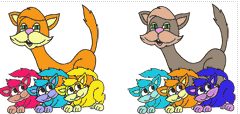# Review Your Numbers: 2, 3 and 4

In this worksheet, students attempt a range of questions that allow them to practise what they have learned so far about addition, subtractions, multiplication and divisionKey stage:  KS 2

Curriculum topic:   Number: Multiplication and Division

Curriculum subtopic:   Solve Multiplication/Division Problems

Popular topics:   Ratio worksheets

Difficulty level:#### Worksheet Overview

In this activity, we will be solving multiplication and division problems based on pictures.

Look at the pictures below, they show a story.There were 4 Cats. Each cat had 3 Kittens.

The first picture shows us four cats.

In the second picture, we can see that each cat now has 3 kittens.

How many kittens altogether?

We can find the total number of kittens either with repeated addition: 3 + 3 + 3 + 3 = 12

Or, we could use the times tables fact 4 × 3 = 12

Both these calculations show the total number of kittens.

Let's try an example question together.

Example

Here are two catsEach cat has 3 kittens.How many kittens are there altogether?

We can find the total number of kittens either with repeated addition: 3 + 3 = 6

Or, we could use the times tables fact 2 × 3 = 6

Both these calculations show the total number of kittens is 6.

Now it's your turn to try some similar questions. Good luck!

### What is EdPlace?

We're your National Curriculum aligned online education content provider helping each child succeed in English, maths and science from year 1 to GCSE. With an EdPlace account you’ll be able to track and measure progress, helping each child achieve their best. We build confidence and attainment by personalising each child’s learning at a level that suits them.

Get started